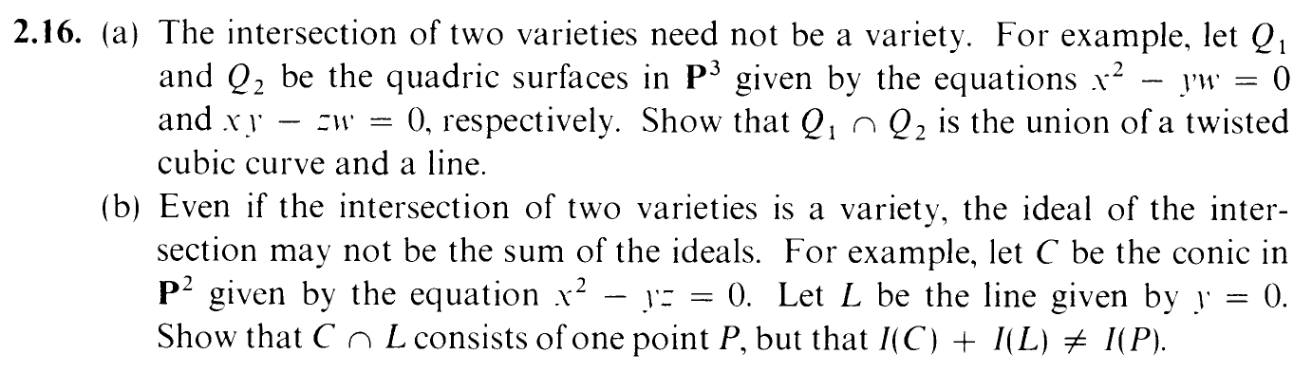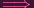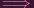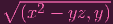I.2.16

3/10/2021Oh, twisted cubic, you appear to be too useful a source of examples in the world of algebraic geometry to dismiss. Alas, I must dance with you if I want to do algebraic geometry. One day, I'll look back at 2.12d and figure out what went so horribly wrong there. I'm not ready yet. But maybe one day...

By the way, this is the second last exercise of this section. Whew. Almost one and a half months since I started this section. What a slowpoke I am, huh? I-I just like taking my time, that's all.

Both parts encompass easy to make misconceptions, things that I would be tempted to conclude under tense circumstances, and provide elegant counterexamples to those. Okay, I'm interested. Let's go.

PART (a):

Now, going off last time, I noted that for the purposes of the surface Q, we're using the coordinate ring k[w,x,y,z], whereas the Wikipedia page (as well as my 2.12d work) for the twisted cubic curve uses the coordinate ring k[x,y,z,w]. Thus, I'll have to translate the Wikipedia page's generators F0,F1,F2, into our new coordinates. Here's the version with new coordinates:

 F0 = wy - x2 F1 = xz - y2 F2 = wz - xy

This exercise is asking us to compare the twisted cubic curve T = Z(F0,F1,F2) and some currently unknown line L with Q1 Q2 = Z(F0,F2)

Now give me a point P = (W,X,Y,Z) Q1 Q2. Right off the bat, we know that

 F0(P) = 0 (1) F2(P) = 0 (2)

Now, if F1(P) = 0, as well, then we know that P T, so that's nice. But what if F1(P)0? Well in that case, it better be in that unknown line.

Specifically,

 F1(P) ≠0F1(P) = a (For some nonzero a)XZ - Y 2 = aXZ = Y 2 + aWXZ = WY 2 + Wa (yep, I multiplied by W on both sides on the basis of "All the variables except W are here, so I guess I'll just add it to the party". Sometimes stupid intuition can take you places)(WZ)X = (WY )Y + Wa(XY )X = (X2)Y + Wa (thanks to (1) and (2))X2Y = X2Y + WaWa = 0W = 0 (Since k is an integral domain and a≠0 by assumption)

So W = 0. But if that's true, then (1) tells us that X = 0

So W = 0,X = 0. Sound familiar? It's a TYPE K line ! (where m = 0). So our L is actually L = Z(w,x) And hence, we've shown that Q1 Q2 T L. And the reverse inclusion is obvious, so we're DUN.

PART (b):
Repasting the exercise for reference:Okay, C = Z(x2 - yz) and L = Z(y).

So if I'm given a P = (X,Y,Z) C L (note we're in P2 now, lol. Almost didn't notice that and caused horror for myself), then right off the bat, the fact that it's in L tells me that Y = 0. But now that I know Y = 0, the fact that it's in C tells me that X = 0. Hence, P = (0, 0,Z) = (0, 0, 1). So C L is the singleton {P}

Now, I(P) = (x,y). And I(C) + I(L) = (x2 - yz,y). Clearly, x is in the former ideal but not in the latter. So they can't be equal. DUN. (btw, this implies that= (x,y), I think)

BTW: A little errata for ya: Back in
2.15b , I said this:

Rank 2 is the 2 dimensional case (the lines coincide), rank 3 is the 1D case (singleton), rank 4 is the 0D case (empty set).

I am one off on every count. Rank 2 is 1D, rank 3 is 0D, and rank 4 is an empty set, which arguably you can't really give a "dimension" to. Doesn't affect the rest of the proof, afaik.